# 20 essential Excel functions (+ examples of use)Excel is a software published by Microsoft and which can be seen compatible with a very wide spectrum of activity sectors. It is ideal for the accounting of a company or the performance analysis of a sales team.

In other words, this software represents a perfect solution for companies wanting a practical tool for their databases, their management and the realization of any commercial reporting. Whatever the core business of your business, here are 20 practical and essential functions or formulas to use Excel correctly.

## Practical Excel functions explained

The range of functions in Excel is very large, and Microsoft provides them as categories to help you differentiate them, depending on your usage needs. You can also go directly to each section:

### Mathematical and trigonometric functions

#### The SUM function

The SUM function is to add a set of data over a range of cells. It is written as follows: SUM = (A1; A2; A3). To make it easier to write, Excel suggests selecting a range of cells at its ends, rather than enumerating all of the data that compose it, as the following example shows: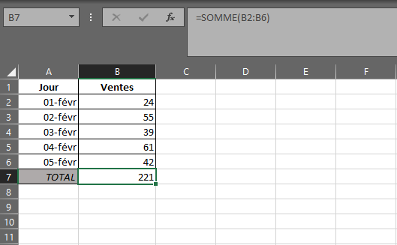#### The SUM.SI function

The SUM.SI function remains very similar to the SUM function, but differs from it on one point: the “.SI” which concludes the title takes into account a criterion in the addition made. In the example below, the criterion makes it possible to add only daily sales of less than 45 units.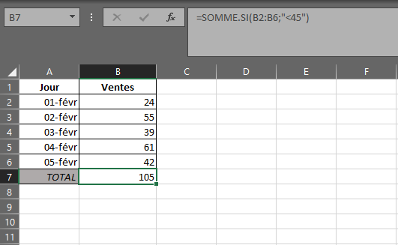### Logic functions

#### The IF function

The IF function poses a condition to be respected. It is one of the most used Excel formulas because it applies to many areas, and intertwines with many other formulas. It is written as follows: = IF (logical test; Value if true; Value if false). The value if false is optional.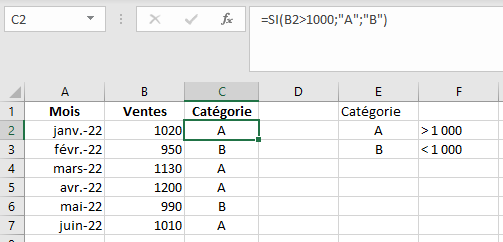#### The AND function

The AND function allows you to group several conditions that must be true within a formula. In this example, it is used in combination with the SI formula: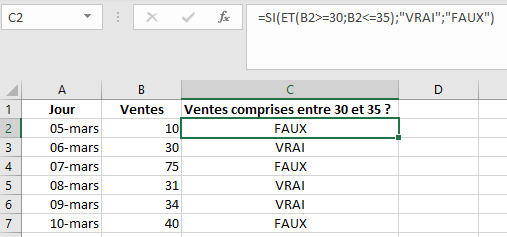#### The OR function

The OR function works similarly to the AND function, but without obligation of truth of several conditions. It suffices that one of the conditions is fulfilled.

In the example below, we consider a normal sale day to be between 20 and 60. Any lower or higher sale will be considered abnormal: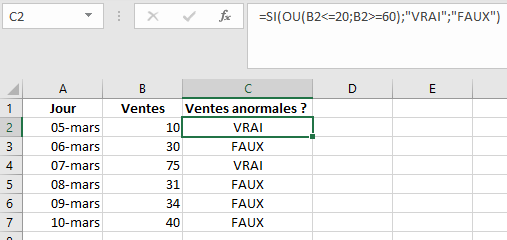#### The NB function

The count function counts numeric values ​​within a defined range, whether it is a cell, row, or column.

In this example, the NB function is used to count the number of days each employee works. Empty cells are excluded from the count.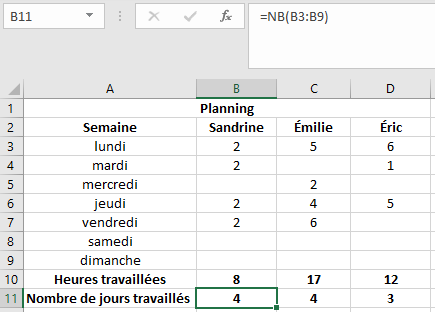### Statistics functions

#### The NB.SI function

The NB.SI function is similar to SOMME.SI in principle, but does not have the same objective. It consists of taking into account a range of data and studying it through the prism of a criterion, as indicated by the “.SI” which composes it. However, it only comes “to count” and not to add the values ​​corresponding to the criterion. Here is an example with the counting of the number of days when the objective of 45 units sold is reached: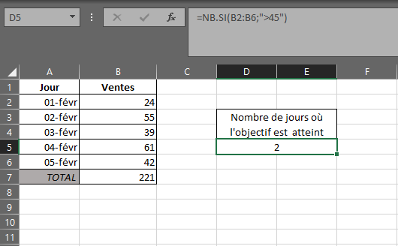#### The EMPTY COUNT function

Just like the NB.SI function, the NB.VIDE function counts a quantity of data according to a predetermined criterion. Here, the criterion is imposed on the Excel user, since the function, in essence, counts the number of empty cells in the range. Here is an example: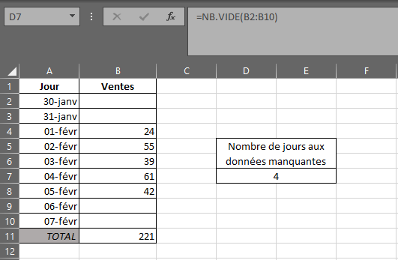#### The AVERAGE function

The AVERAGE function, as the name suggests, is knowing the average of values ​​over a range of data. Just like the SUM function, its result is automatically suggested at the bottom right of the interface, alongside the number of nonblank cells when the range is selected. Its calculation method is therefore strictly similar to SUM, and here is an example: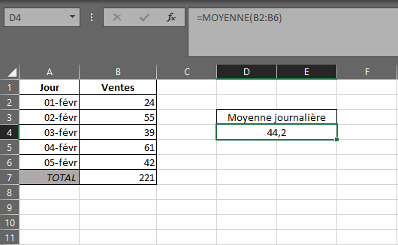### Date and time functions

#### The YEAR function

The date and time functions make it possible to quickly find out and isolate information from a date data item for subsequent analysis using other functions. Among them, you can notably find the YEAR function. It provides for a cell the year corresponding to the entered date. Here is an example :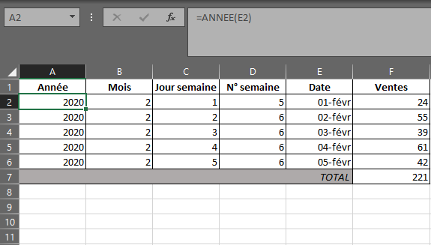#### The MONTH function

As explained previously, the MONTH function is very similar to YEAR and consists in isolating the number of the month in a date. His writing remains the same: = MONTH (A1). Here is an example :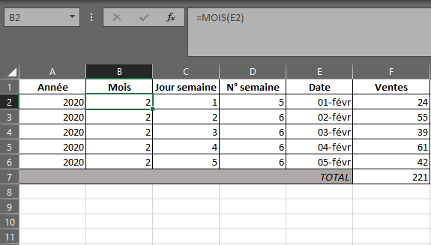#### The DAY function

Once again, this function has a very basic writing: = DAY (A1). Here, the function isolates the number of the day from the date analyzed. This can come in handy in a larger database to understand if an activity is peaking during a given time period every month. Here is an application of this formula: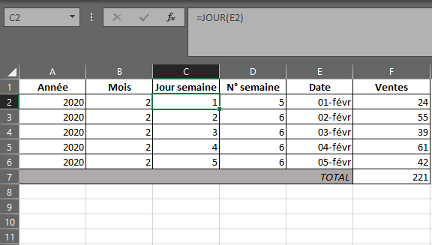#### The WEEK NO. Function

Unlike other functions of its kind, the WEEK NO. Function does not isolate a data component of a date, but takes advantage of it to create another: the number of the week. Its principle remains strictly the same in terms of drafting: = WEEK NO. (A1). Here is an example :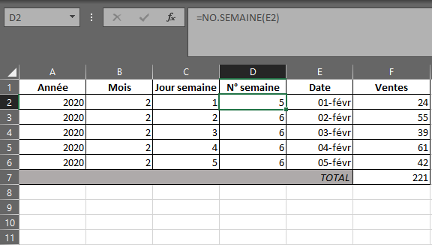### Text functions

#### The SUBSTITUTE function

Excel suggests replacing portions of text in other cells with the SUBSTITUTE function. It is very practical in long strings of characters where it is imperative to replace a portion by another string of characters. Taking into account the existing text, the section to be replaced and the replacing section, here is how this formula is applied: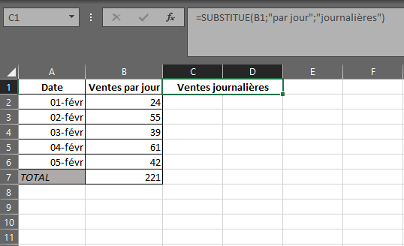#### The REPLACE function

The REPLACE function, contrary to appearances, differs from the SUBSTITUTE function. The latter replaces in a character string all the occurrences of the same series of characters. REPLACE is based rather on a location selection in the character string to replace a targeted portion or occurrence. This can be useful in the case of a code format that has not been accepted by Excel. Here is an example: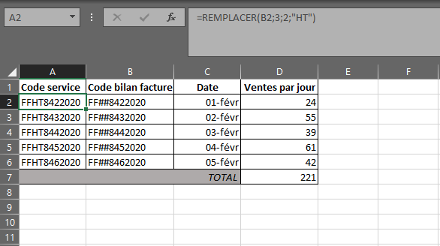#### The NBCAR function

The NBCAR function consists of counting the number of characters within the same string. This is very useful for categorizing information, or even, in the case of SEO and a list of title tags, to know the number of characters in each of them. Here is an example :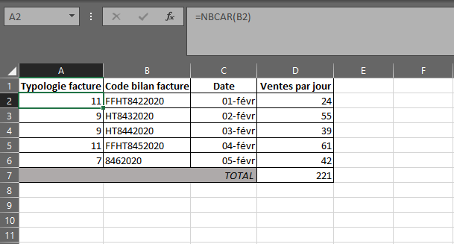### Search and reference functions

#### The SEARCH function

The SEARCH function consists of going to parallel several ranges to find the value of a cell linked to another. In the following example, suppose that the lowest number of sales is known, but not the corresponding type of invoice in a database that is much larger and more difficult to process. To do this, the SEARCH function finds this value (i.e. 24), recall the list of values ​​that it includes (D2 to D6) and gives a list of parallel values ​​(A2 to A6) to find the one linked to the 24 daily sales ( 11).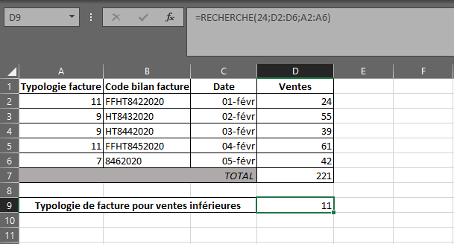#### The HLOOKUP function

The HLOOKUP function wants to be different from its colleague, since it is based on a “horizontal” search, in other words by taking into consideration the lines of the spreadsheet. In this example, the formula targets the title “Sales” and the selection of the range D1 to D6 by asking for the value included in line 4 of this same range.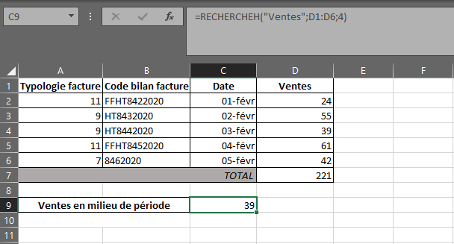#### The VLOOKUP function

Finally, the VLOOKUP function is used to isolate an element and find the one that corresponds to it in a large range of data, on the basis of a so-called “vertical” search. Here, the objective is to know the sales for invoice n ° 7 over the period from February 1 to 5, 2020.

The function takes into account the value 7 in A10 to find it in the large selection of data A2 to D6, by supplying the corresponding value in column 4, that is to say in D. The FALSE which ends the formula makes it possible to impose on the function to provide an exact value, i.e. 42.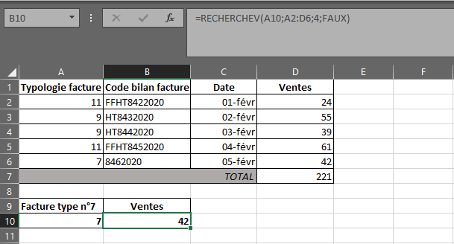#### The XLOOKUP function

Microsoft wanted to simplify the use of its Excel software by providing a new version of the LOOKUP functions: the XLOOKUP function. Combining all the advantages of its counterparts, it simplifies the selection of data for the same result, sparing certain ancillary calculations. Here is an example :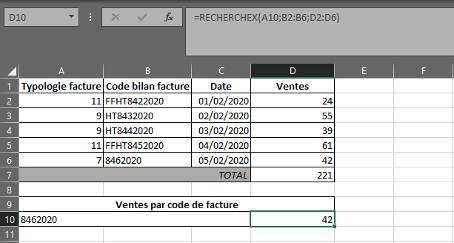There is always a good reason to use Excel. Tables, invoices, receipts, schedules: find out these excel chart templates to format your data and highlight your results.